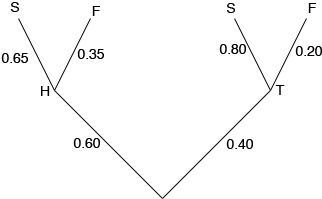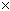SEARCH HOMEMath Central Quandaries & QueriesQuestion from cookie, a student: You wish to open a new restaurant and are considering locations in Toronto and Hamilton, but only one location will actually become available. If it is built in Toronto, the restaurant stands an 80% chance of successfully surviving its first year. However, if it is built in Hamilton, its chance of survival falls to 65%. It is estimated that the chance of Hamilton being available is 60% and Toronto being available is 40%. Find the probability that the restaurant will : a) survive its first year b) be built in Toronto and survive its first year c) be built in Hamilton, given that it survived its first year d) not survive its first year, given that it is built in Toronto ThanksI would use a tree diagram to solve this problem. In my diagram H is Hamilton, T is Toronto, S is success and F is failure.The numbers beside the branches are probabilities, so on the Hamilton side 0.60 is the probability that the location in Hamilton becomes available, 0.65 is the probability that the restaurant survives the first year if it is in Hamilton and hence 1 - 0.65 = 0.35 is the probability it fails if it is in Hamilton. You can now calculate the probability of each path through the tree from bottom to top. For example the probability that the location in Hamilton becomes available and the restaurant fail is 0.600.35 = 0.21 .

Calculate the probability of each path through the tree and then see if these probabilities help you find what is asked for in a), b), c) and d).

PennyMath Central is supported by the University of Regina and The Pacific Institute for the Mathematical Sciences.